# Quiz 11: Investment Planning

a) Company name: CC Company Exchange: NYSE b) Current market price of stock: \$41.63 per share Market stock price 1 year ago: \$38 per share Market stock price 3 years ago: \$33 per share Market stock price 5 years ago: \$25 per share Calculate the percentage price change using the following formula, which is as follows;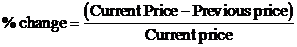The following table G1 shows the percentage change of the market stock for the given information, which is as follows: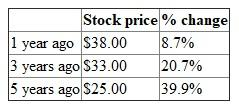c) Its headquarters is at in A, G state. Its Chairman of the Board and CEO is M K. 5% of shares are held by insiders. d) The CC Company mission is to refresh the world, to inspire moments of optimism and happiness, and to create value and make a difference. Its main products are sugary, carbonated drinks such as C-C, S and F. e) The CC drink was created in 1886 and patented in 1887. In 1899, The CC Company started franchising bottling operations in the U S. f)CC's competitors include Dr. P S Group, Inc. , N and P, Inc. g) Sales and profit summaries can be found on their Income Statement. Their sales for 2013 were \$46,854,000,000. Their profit for 2013 was \$8,584,000,000. h) the following table G2, shows the financial ratios and measures of the company CC, which is as follows: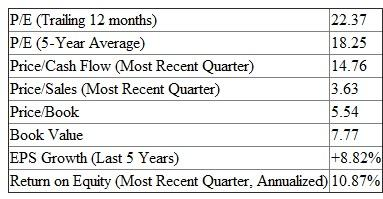i) A recent development is that The CC Company paid \$2.2 billion for 17% of Monster Beverage. The CEO of The CC Company, M K, acknowledged that the company's 2013 volume and revenue results did not meet expectations. The company has plans to accelerate growth, expand their profitable portfolio, maximize productivity, win at the point of sale and invest in the next generation of leaders. Based on my findings, it can be suggestible for the company for an future investment. Its EPS Growth and Return on Equity look good. Although sales were slow in 2013, there are plans to grow. On the downside, it's possible sugary drinks will soon be a thing of the past as consumers see it as a cause of obesity.
1) The following table A1 shows the rework on the excel sheet 11.1 (Determining amount of invested capital). In the following table A1 only Part A of the worksheet needs to be used. Consider that the first child will start college in 6 years' time. The worksheet will be as follows: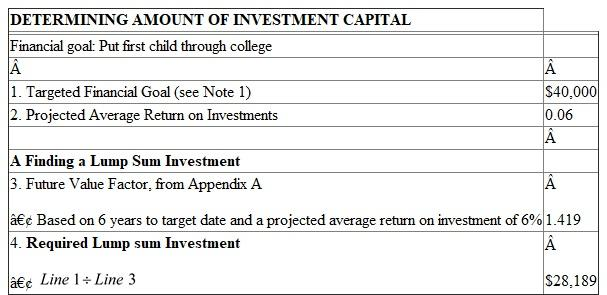In the table A1 the required lump sum investment is calculated by multiplying the targeted financial goal.i.e. line 1 and future value factor.i.e. line 3. In the table A1 , assuming that the first child requires all \$40,000 at the start of college, there is enough to send the child to college, if Persons G and SU have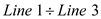right now. The following table A2 shows the rework on the excel sheet 11.1 (Determining amount of invested capital). In table A2 consider that the second child will start college in 8 years' time. The worksheet will be as follows: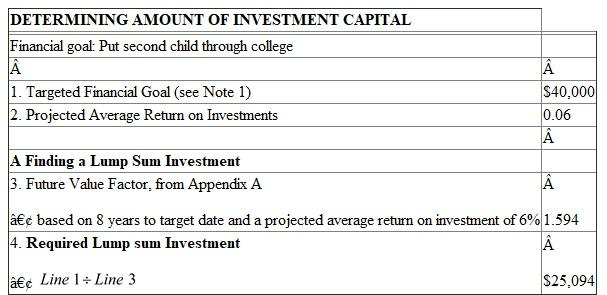In the table A2 the required lump sum investment is calculated by multiplying the targeted financial goal.i.e. line 1 and future value factor.i.e. line 3. Assuming that the child requires all \$40,000 at the start of college , there is enough to send the child to college, if Persons G and SU haveright now. The total they will need to send both children to college is calculated as follows: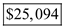Therefore, from the above calculations it can be concluded that the \$45,000 they have is not enough, they need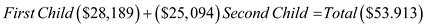2) The following table B1 shows the rework on the excel sheet 11.1 (Determining amount of invested capital). Here, as it is stated to know the investment worth, the following table B1 , shows the rework of the excel sheet 11.1 only Part B of the worksheet needs to be used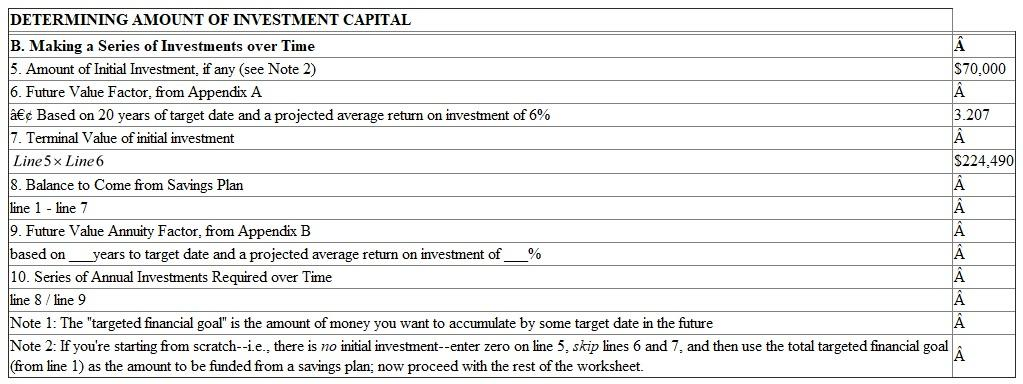In the table B1 the value of initial investment is calculated by multiplying initial amount.i.e. line 5 with future value factor.i.e. line 6. From the above table it can be concluded that, in 20 years , the investments will be worth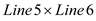3) Use the following formula of future value of annuity , here, in order to obtain the investment worth after 20 years at 6 percent interest , which is as follows: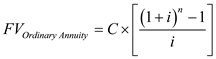The variables are as follows:Substitute \$6,000 for ' C ' 0.06 percent for '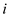' and 20 years for ' n '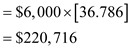Therefore, from the above calculation it can be concluded that the future value of the investment will be worth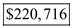4) Since Person S thinks they will be able to put away more after the children are out of school, they can use part of the \$50,000 that they received from an inheritance to fund the children's college education. The amount they will need is the difference between the \$53,913 from part (a) and the \$45,000 they already have. This difference is \$8,913. After the children are out of school, they can save more towards their retirement.